# Norm (mathematics) facts for kids

Kids Encyclopedia Facts

In mathematics, the norm of a vector is its length. A vector is a mathematical object that has a size, called the magnitude, and a direction. For the real numbers, the only norm is the absolute value. For spaces with more dimensions, the norm can be any function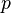$p$ with the following three properties:

1. Scales for real numbers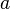$a$, that is,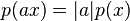$p(ax) = |a|p(x)$.
2. Function of sum is less than sum of functions, that is,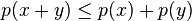$p(x + y) \leq p(x) + p(y)$ (also known as the triangle inequality).
3.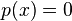$p(x) = 0$ if and only if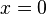$x = 0$.

## Definition

For a vector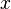$x$, the associated norm is written as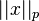$||x||_p$, or L$p$ where$p$ is some value. The value of the norm of$x$ with some length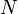$N$ is as follows: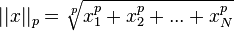$||x||_p = \sqrt[p]{x_1^p+x_2^p+...+x_N^p}$

The most common usage of this is the Euclidean norm, also called the standard distance formula.

## Examples

1. The one-norm is the sum of absolute values: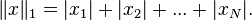$\|x\|_1 = |x_1| + |x_2| + ... + |x_N|.$ This is like finding the distance from one place on a grid to another by summing together the distances in all directions the grid goes; see Manhattan Distance.
2. Euclidean norm (also called L2-norm) is the sum of the squares of the values: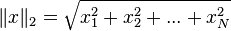$\|x\|_2 = \sqrt{x_1^2 + x_2^2 + ... + x_N^2}$
3. Maximum norm is the maximum absolute value: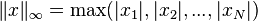$\|x\|_{\infty} = \max(|x_1|,|x_2|,...,|x_N|)$
4. When applied to matrices, the Euclidean norm is referred to as the Frobenius norm.
5. L0 norm is the number of non-zero elements present in a vector.

## Related pagesNorm (mathematics) Facts for Kids. Kiddle Encyclopedia.